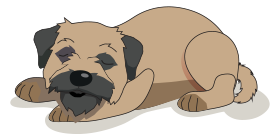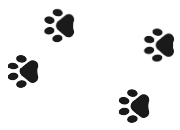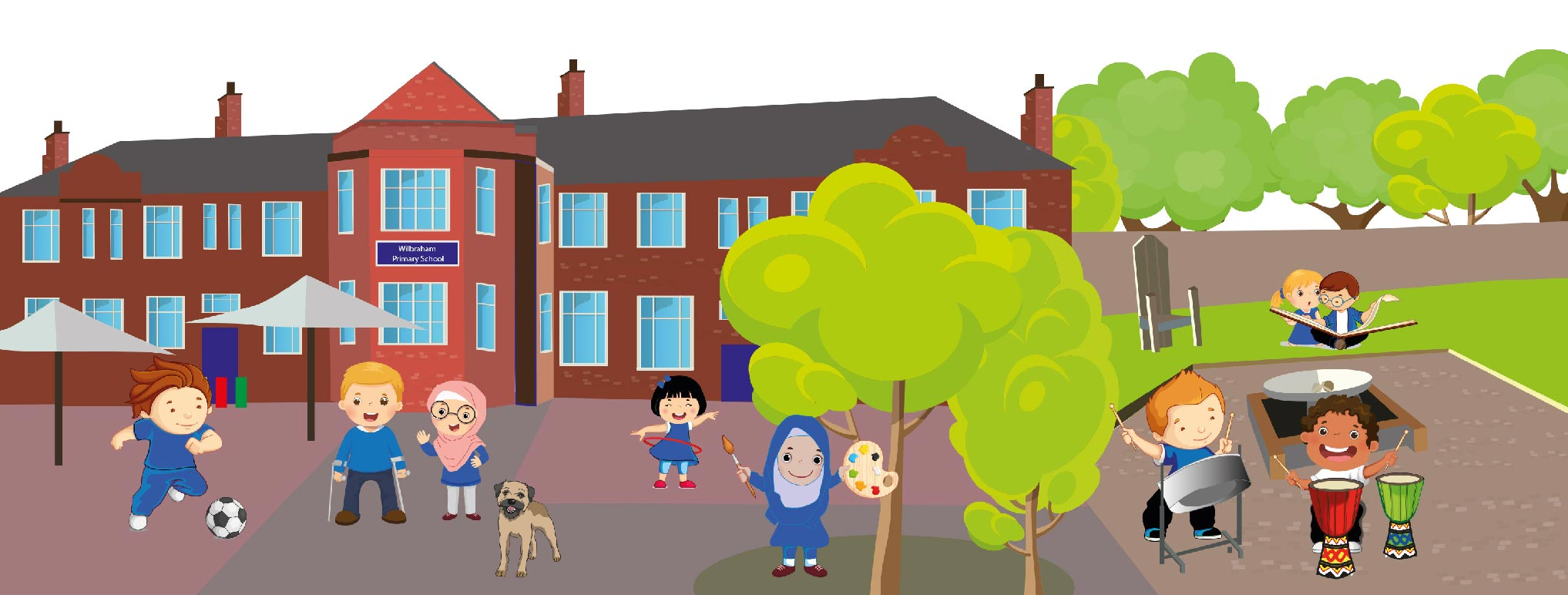# Mental Calculations

​Mental strategies do not only refer to calculations done in your head.  At Wilbraham Primary School we teach several ways of carrying out mental calculations, including both working in your head and making jottings using pencil and paper.

As children move through the school, their calculation methods become increasingly efficient. Often, the fastest way to carry out a calculation is to use a mental strategy.
Consider 1999+5=

Rather than adding this using the formal written method (in columns), it is easier to partition the 5 into 1 and 4. The calculation then becomes:
1999+1+4=2000+4=2004

The ability to calculate in your head is an important part of Mathematics. Children need to develop a range of mental strategies. These will then help your child to quickly work out new facts from related facts. Learning number bonds in their early years and later on learning their tables are important, as these will help the children become faster at mental calculations. Once they know these, they are able to find further related facts.

We teach a range of strategies to encourage children to investigate and find efficient ways of calculating mentally. These include:
Using a number line (see the help pages on Mental Calculations and Jotting)
The ways in which children use the number line and the complexity of calculations depends on the stage at which they are at.

### RaPa CoDa numBo

• Ra- This involves rounding and adjusting

458+17 becomes

458+2+15=460+15

• Pa- This involves partitioning

327+22 becomes

327+20+2

• Co- This involves counting

865-48 Count back 4 tens and 8 ones.

• Da-This involves looking for doubles

34+38 becomes

Double 34 (68) add the extra 4

• NumBo- This involves using their number bonds.

Number bonds up to 10: 5+5, 6+3 8-4

Number bonds up to 20: 15+3, 14-6, 13+7

Multiples of ten to 100: 50+60, 80+20, 50-30

Number bonds up to 100: 24+32, 55+45, 82-11

​Additional help sheets are listed below:

Wilbraham Primary School

Platt Lane,
Fallowfield,
Manchester, M14 7EB

0161 224 3900